Win up to 100% scholarship on Aakash BYJU'S JEE/NEET courses with ABNAT Win up to 100% scholarship on Aakash BYJU'S JEE/NEET courses with ABNAT

# Coulomb's Law

## What Is Coulomb’s Law?

According to Coulomb’s law, the force of attraction or repulsion between two charged bodies is directly proportional to the product of their charges and inversely proportional to the square of the distance between them. It acts along the line joining the two charges considered to be point charges.

## Coulomb’s Law Formula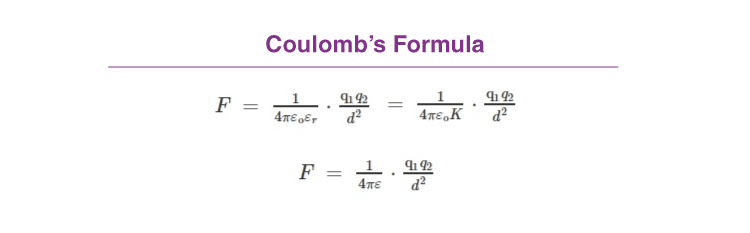In short, F ∝ q1q2/d2

Where,

• ε is absolute permittivity,
• K or εr is the relative permittivity or specific inductive capacity
• ε0 is the permittivity of free space.
• K or εr is also called a dielectric constant of the medium in which the two charges are placed.

### History of Coulomb’s Law

In 1785, French physicist Charles Augustin de Coulomb coined a tangible relationship in mathematical form between two bodies that have been electrically charged. He published an equation for the force causing the bodies to attract or repel each other, which is known as Coulomb’s law or Coulomb’s inverse-square law.

## Coulomb’s Law in Vector Form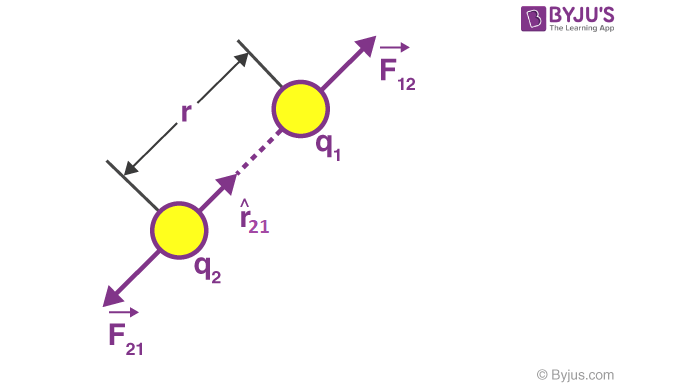$$\begin{array}{l}{{\vec{F}}_{12}}=\frac{1}{4\pi {{\in }_{0}}}\frac{{{q}_{1}}{{q}_{2}}}{r_{12}^{2}}{{\hat{r}}_{12}}; \;\;{{\vec{F}}_{12}}=-{{\vec{F}}_{21}}\end{array}$$

Here, F12 is the force exerted by q1 on q2, and F21 is the force exerted by q2 on q1.

Coulomb’s law holds for stationary charges only, which are point sized. This law obeys Newton’s third law

$$\begin{array}{l}\left( ie\,\,\,{{{\vec{F}}}_{12}}=-{{{\vec{F}}}_{21}} \right)\end{array}$$

Force on a charged particle due to a number of point charges is the resultant of forces due to individual point charges, i.e.,

$$\begin{array}{l}\vec{F}={{\vec{F}}_{1}}+{{\vec{F}}_{2}}+{{\vec{F}}_{3}}+……\end{array}$$

## What Is 1 Coulomb of Charge?

A Coulomb is a charge which repels an equal charge of the same sign with a force of 9×109 N when the charges are one metre apart in a vacuum. Coulomb force is the conservative mutual and internal force.

The value of εo is 8.86 × 10-12 C2/Nm2 (or) 8.86 × 10-12 Fm–1

Note: Coulomb force is true only for static charges.

## Coulomb’s Law – Conditions for Stability

If q is slightly displaced towards A, FA increases in magnitude while FB decreases in magnitude. Now, the net force on q is toward A, so it will not return to its original position. So for axial displacement, the equilibrium is unstable.

If q is displaced perpendicular to AB, the force FA and FB bring the charge to its original position. So, for perpendicular displacement, the equilibrium is stable.

## Key Points on Coulomb’s Law

1. If the force between two charges in two different media is the same for different separations,

$$\begin{array}{l}F=\frac{1}{K}\frac{1}{4\pi {{\in }_{0}}}\frac{{{q}_{1}}{{q}_{2}}}{{{r}^{2}}} = constant\end{array}$$

2.  Kr2 = constant or K1r12 = K2r2

3. If the force between two charges separated by a distance ‘r0’ in a vacuum is the same as the force between the same charges separated by a distance ‘r’ in a medium, then from Coulomb’s law, Kr2 = r02

4. Two identical conductors having charges q1 and q2 are put to contact and then separated, after which each will have a charge equal to (q1 + q2)/2. If the charges are q1 and –q2, each will have a charge equal to (q1 – q2)/2.

5. Two spherical conductors having charges q1 and q2 and radii r1 and r2 are put to contact, and then separated the charges of the conductors after contact is

q1 = [r1/(r1 + r2)] (q1 + q2) and q2 = [r2/(r1 + r2)] (q1 + q2)

6. If the force of attraction or repulsion between two identical conductors having charges q1 and q2 when separated by a distance d is F. Also, if they are put to contact and then separated by the same distance, the new force between them is:

$$\begin{array}{l}F = \frac{F{{\left( {{q}_{1}}+{{q}_{2}} \right)}^{2}}}{4{{q}_{1}}{{q}_{2}}}\end{array}$$

7. If charges are q1 and -q2, then F = F(q1 + q2)2 / 4q1q2

8. Between two electrons separated by a certain distance: Electrical force/Gravitational force = 1042

9. Between two protons separated by a certain distance: Electrical force/Gravitational force = 1036

10. Between a proton and an electron separated by a certain distance: Electrical force/Gravitational force = 1039

11. The relationship between the velocity of light, the permeability of free space and the permittivity of free space is given by the expression c = 1 / √ (μoεo )

12. If Coulomb’s law is applied to two identical balls of mass m are hung by silk thread of length ‘l’ from the same hook and carry similar charges q, then,

• The distance between balls
$$\begin{array}{l}=[\frac{q^{2}2l }{4\pi \epsilon _{o}mg}]^{\frac{1}{3}}\end{array}$$
• The tension in the thread
$$\begin{array}{l}=\sqrt{f^2+(mg)^2}\end{array}$$
• If the total system is kept in space, then the angle between threads is 180°, and tension in a thread is given by
$$\begin{array}{l}T=\frac{1}{4\pi {{\in }_{0}}}\frac{{{q}^{2}}}{4{{\ell }^{2}}}\end{array}$$
• A charge Q is divided into q and (Q – q). Then, the electrostatic force between them is maximum when
$$\begin{array}{l}\frac{q}{Q}=\frac{1}{2}\;\ (or) \;\;\;\frac{q}{\left( Q-q \right)}=1\end{array}$$

## Limitations of Coulomb’s Law

• The law is applicable only for the point charges at rest.
• Coulomb’s law can only be applied in those cases where the inverse square law is obeyed.
• It is difficult to implement Coulomb’s law where charges are in arbitrary shape because, in such cases, we cannot determine the distance between the charges.
• The law can’t be used directly to calculate the charge on big planets.

### Relative Permittivity of a Material

$$\begin{array}{l}{{\varepsilon }_{r}}=K=\frac{force\;between\;two\;charge\;in\;air}{force\;between\;same\;charge\;in\;the\;medium\;at\;the\;same\;distance}\end{array}$$
.

$$\begin{array}{l}{{\varepsilon }_{r}}=\frac{F_a}{F_m}\end{array}$$
• For air K = 1
• For metals, K = infinity

The force between 2 charges depends on the nature of the intervening medium, whereas gravitational force is independent of the intervening medium.

For air or vacuum,

$$\begin{array}{l}F\text{ }=\text{ }\frac{\text{1}}{\text{4}\pi {{\varepsilon }_{\text{o}}}}.\frac{{{\text{q}}_{\text{1}}}{{q}_{2}}}{{{d}^{2}}}\end{array}$$
since for air or vacuum,
$$\begin{array}{l}{{\varepsilon }_{r}}= K = 1\end{array}$$

The value of 1/4πε0 is equal to 9 × 109 Nm2/C2.

## Application of Coulomb’s Law

• To calculate the distance and force between the two charges
• The electric field can be calculated using Coulomb’s law
$$\begin{array}{l}E = \frac{F}{Q_{T}}\left ( \frac{N}{C} \right )\end{array}$$

Where, E = Strength of the electric field

F = Electrostatic force

QT = Test charge in Coulombs

• To calculate the force on one point due to the presence of several points (Theorem of superposition)

## Problems on Coulomb’s Law

Problem 1: Charges of magnitude 100 microcoulomb each are located in a vacuum at the corners A, B and C of an equilateral triangle measuring 4 meters on each side. If the charge at A and C are positive and the charge at B negative, what is the magnitude and direction of the total force on the charge at C?

Sol.

The situation is shown in the figure below. Let us consider the forces acting on C due to A and B.

Now, from Coulomb’s law, the force of repulsion on C due to A, i.e., FCA in the direction of AC, is given by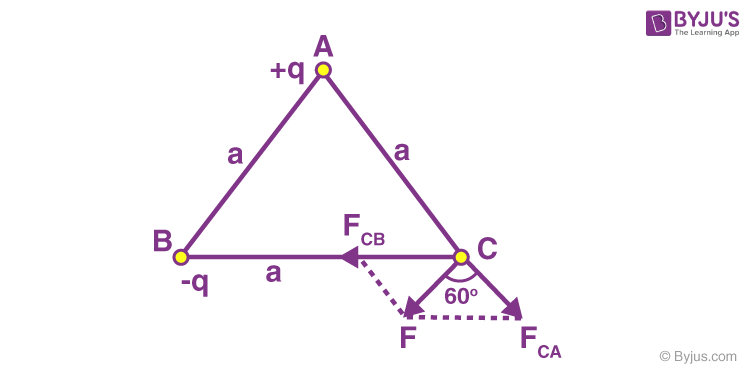$$\begin{array}{l}F_{CA} =\frac{1}{4\pi {{\varepsilon }_{0}}}. \frac{q\times q}{{{a}^{2}}}\end{array}$$
along AC

The force of attraction on C due to B, i.e., FCB in direction CB, is given by

$$\begin{array}{l}F_{CB}= \frac{1}{4\pi {{\varepsilon }_{0}}}. \frac{q\times q}{{{a}^{2}}}\end{array}$$
along CB

Thus, the two forces are equal in magnitude. The angle between them is 120º. The resultant force F is given by

$$\begin{array}{l}F =\sqrt{{{F}^{2}_{C{{A}}}}+{{F}^{2}_{C{{B}}}}+2{{F}_{CA}}\times {{F}_{CB}}\cos 120{}^\text{o}}\end{array}$$
$$\begin{array}{l}=\frac{{{q}^{2}}}{4\pi ε{{a}^{2}}} \\= \frac{9\times {{10}^{9}}\times {{(100\times {{10}^{-6}})}^{2}}}{{{4}^{2}}}\end{array}$$
= 5.625 Newton

This force is parallel to AB.

Problem 2: The negative point charges of unit magnitude and a positive point charge q are placed along the straight line. At what position and for what value of q will the system be in equilibrium? Check whether it is stable, unstable or neutral equilibrium.

Sol.

The two negative charges – A and B – of unit magnitude are shown in the figure below. Let the positive charge q be at a distance rA from A and at a distance rB from B.

Now, from Coulomb’s law, force on q due to A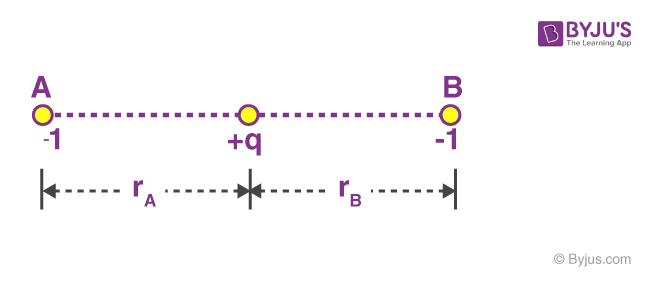$$\begin{array}{l}F_{qA}=\frac{1}{4\pi{{\varepsilon}_{0}}}.\frac{q}{{{r}_{A}}^{2}}\ towards\ A\end{array}$$

Force on q due to B

$$\begin{array}{l}F_{qB}=\frac{1}{4\pi{{\varepsilon}_{0}}}. \frac{q}{{{r}_{B}}^{2}}\ towards\ B\end{array}$$
.

These two forces acting on q are opposite and collinear. For the equilibrium of q, the two forces must also be equal, i.e.,

|FqA| = |FqB|

or

$$\begin{array}{l}\frac{1}{4\pi {{\varepsilon }_{0}}}. \frac{q}{{{r}_{A}}^{2}} = \frac{1}{4\pi {{\varepsilon }_{0}}}. \frac{q}{{{r}_{B}}^{2}}\end{array}$$

Hence, rA = rB

So, for the equilibrium of q, it must be equidistant from A & B, i.e., in the middle of AB.

Now for the equilibrium of the system, A and B must be in equilibrium.

For the equilibrium of A,

Force on A by q

$$\begin{array}{l}=\frac{1}{4\pi {{\varepsilon }_{0}}}. \frac{q}{{{r}_{A}}^{2}}\end{array}$$
towards q

Force on A by B

$$\begin{array}{l}=\frac{1}{4\pi {{\varepsilon }_{0}}}. \frac{(1)(1)}{{{({{r}_{A}}+{{r}_{B}})}^{2}}}\end{array}$$
$$\begin{array}{l}=\frac{1}{4\pi {{\varepsilon }_{0}}}. \frac{1}{{{(2{{r}_{A}})}^{2}}}\end{array}$$
away from q

The two forces are opposite and collinear. For equilibrium, the forces must be equal, opposite and collinear. Hence,

$$\begin{array}{l}\frac{1}{4\pi {{\varepsilon }_{0}}}. \frac{q}{{{r}_{A}}^{2}}= \frac{1}{4\pi {{\varepsilon }_{0}}}. \frac{1}{{{(2{{r}_{A}})}^{2}}}\end{array}$$

or q = 1/4 in the magnitude of either charge

It can also be shown that for the equilibrium of B, the magnitude of q must be 1/4 of the magnitude of either charge.

Problem 3: A positive charge of 6×10-6 C is 0.040m from the second positive charge of 4×10-6 C. Calculate the force between the charges.

Given

q1 =  6×10-6 C

q= 4×10-6 C

r = 0.040 m

Sol.

$$\begin{array}{l}F_{e} = k \frac{q_{1}q_{2}}{r^{2}}\end{array}$$

$$\begin{array}{l}F_{e} = \frac{8.99\times 10^{9}\left ( 6\times 10^{-6} \right )\left (4\times 10^{-6} \right )}{\left ( 0.04^{2} \right )}\end{array}$$

$$\begin{array}{l}F_{e} = \frac{8.99\times 10^{9}\left ( 2.4\times 10^{-11} \right )}{1.6\times 10^{-3}}\end{array}$$

$$\begin{array}{l}F_{e} = \frac{0.21576}{1.6\times 10^{-3}}\end{array}$$

F= 134.85 N

Problem 4: Two-point charges, q= +9 μC and q= 4 μC, are separated by a distance r = 12 cm. What is the magnitude of the electric force?

given

k = 8.988 x 109 Nm2C−2

q= 9 ×10-6 C

q2 = 4 ×10-6 C

r = 12cm = 0.12 m

Sol:

$$\begin{array}{l}F_{e} = k \frac{q_{1}q_{2}}{r^{2}}\end{array}$$

$$\begin{array}{l}F_{e} = \frac{8.99\times 10^{9}\left ( 9\times 10^{-6} \right )\left (4\times 10^{-6} \right )}{\left ( 0.12^{2} \right )}\end{array}$$

$$\begin{array}{l}F_{e} = \frac{8.99\times 10^{9}\left ( 3.6\times 10^{-11} \right )}{0.0144}\end{array}$$

$$\begin{array}{l}F_{e} = \frac{0.32364}{0.0144}\end{array}$$

F= 22.475 N

### Coulomb’s Law and Superposition Principle – Electrostatics – JEE Main 2023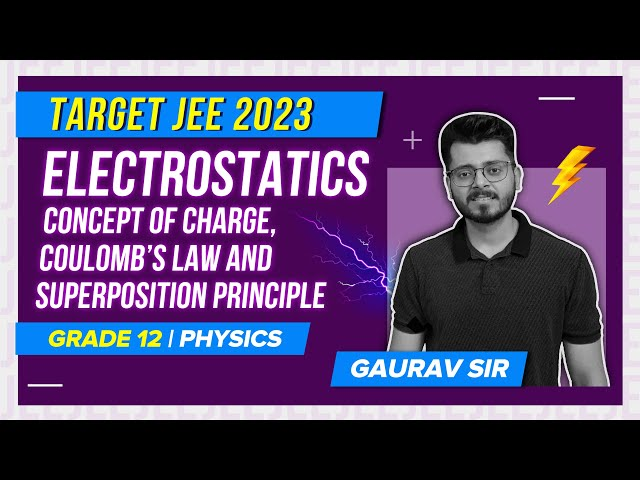### Coulomb’s Law – Concepts and Questions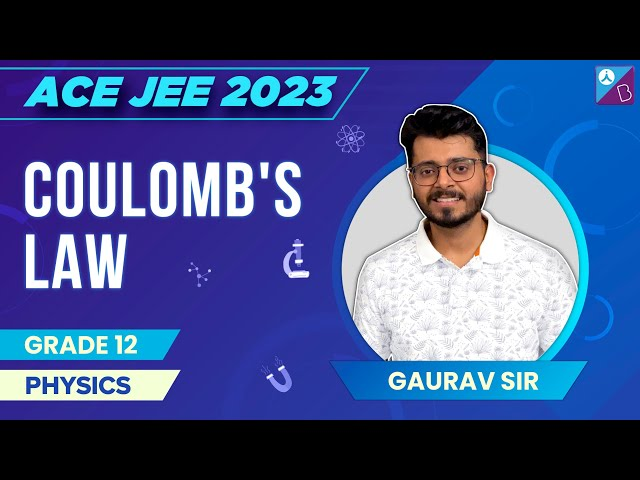## Frequently Asked Questions on Coulomb’s Law

Q1

### State Coulomb’s inverse-square law in electrostatics.

The electrostatic force of attraction or repulsion between two stationary point charges is directly proportional to the product of the magnitudes of the charges and inversely proportional to the square of the distance between them.

Q2

### What are the limitations of Coulomb’s law?

Coulomb’s law holds good for stationary point charges.
Coulomb’s law is not universal, as it depends on the properties of the intervening medium.

Q3

### Is the electrostatic force between two point charges a central force?

Yes. The electrostatic force between two point charges always acts along the line joining the two charges. Hence, it is a central force.

Q4

### What is one Coulomb of charge?

One Coulomb of charge is that charge which, when placed at rest in a vacuum at a distance of one metre from an equal and similar stationary charge, repels it and is repelled by it with a force of 9 x 109 Newton.

Test Your Knowledge on Coulombs Law